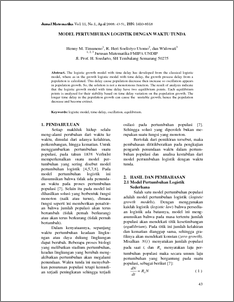# MODEL PERTUMBUHAN LOGISTIK DENGAN WAKTU TUNDA

Henny, Henny and Widowati, Widowati and Robertus, Heri (2008) MODEL PERTUMBUHAN LOGISTIK DENGAN WAKTU TUNDA. JURNAL MATEMATIKA DAN KOMPUTER, 11 (1). pp. 43-51. ISSN 1410-8518Preview
PDF
98Kb

## Abstract

The logistic growth model with time delay has developed from the classical logistic model, where as in the growth logistic model with time delay, the growth process delay from a population is calculated. This delay cause population decrease then increase so oscillation appears in population growth. So, the solution is not a monotonous function. The result of analysis indicate that the logistic growth model with time delay have two equilibrium points. Each equilibrium points is analyzed for their stability based on time delay variation on the population growth. The longer time delay in the population growth can cause the unstable growth, hence the population decrease and become extinct.

Item Type: Article logistic model, time-delay, oscillation, equilibrium. Q Science > Q Science (General) Faculty of Science and Mathematics > Department of Mathematics 1876 INVALID USER 30 Nov 2009 10:01 04 Dec 2009 08:47

Repository Staff Only: item control page